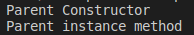# Super Function in Python

## Super Function in Python

In this article, I am going to discuss super Function in Python with examples. Please read our previous article where we discussed Method Resolution Order (MRO) in Python. As part of this article, we are going to discuss the following pointers which are related to Super Function in Python.

1. Super() Function in Python
2. Which scenarios super() function is required?
3. Different Approaches for Calling method of a specific super class.
4. Different cases for super() function
##### Super() Function in Python:

super() is a predefined function in python. By using super() function in child class, we can call,

1. Super class constructor.
2. Super class variables.
3. Super class methods.

Program: Calling super class constructor from child class constructor using super() (demo16.py)

```class A:
def __init__(self):
print("super class A constructor")

class B(A):
def __init__(self):
print("Child class B constructor")
super().__init__()

b=B()```

Output: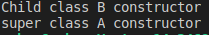##### Which scenarios super() function is required?

When both superclass and child class may have the same method names, same variable names, some scenarios may come where we want to use both of them. In such a case, using the super() function we can call the parent class method.

Program: Calling super class method from child class method using super() (demo17.py)

```class A:
def m1(self):
print("Super class A: m1 method")
class B(A):
def m1(self):
print("Child class B: m1 method")
super().m1()
b=B()
b.m1()```

Output: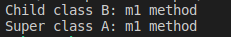Program: Calling super class variable from child class method using super() (demo18.py)

```class A:
x=10
def m1(self):
print("Super class A: m1 method")
class B(A):
x=20
def m1(self):
print('Child class x variable', self.x)
print('Super class x variable', super().x)
b=B()
b.m1()```

Output: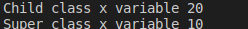###### Program: demo19.py
```class Person:
def __init__(self, name, age):
self.name=name
self.age=age
def display(self):
print('Name:', self.name)
print('Age:', self.age)
class Employee(Person):
def __init__(self, name, age, empno, address):
super().__init__(name, age)
self.empno=empno

def display(self):
super().display()
print('Emp No:', self.empno)

e1=Employee('Neethu',16,111,"Delhi")
e1.display()```

Output: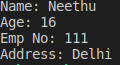##### Different Approaches for Calling method of a specific superclass

We can use the following approaches:

1. NameOfTheClass.methodname(self) – It will call super class method
2. super(NameOfTheClass, self).methodname – It will call the method of super class of mentioned class name.
###### Program: NameOfTheClass.methodname(self) (demo20.py)
```class A:
def m1(self):
print('m1() method from A class')
class B(A):
def m1(self):
print('m1() method from B class')
class C(B):
def m1(self):
print('m1() method from C class')
class D(C):
def m1(self):
print('m1() method from D class')
class E(D):
def m1(self):
A.m1(self)

e=E()
e.m1()```

Output: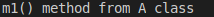###### Program: super(NameOfTheClass, self).methodname (demo21.py)
```class A:
def m1(self):
print('m1() method from A class')
class B(A):
def m1(self):
print('m1() method from B class')
class C(B):
def m1(self):
print('m1() method from C class')
class D(C):
def m1(self):
print('m1() method from D class')
class E(D):
def m1(self):
#A.m1(self)
super(D, self).m1()

e=E()
e.m1()```

Output: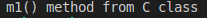Since using this approach, the super class of specified class will be called, class C is called. The mentioned class is D and its super class is C.

##### Different cases for super() function
###### CASE1:

From a child if we want to access the parent class instance variable then we should use a self variable only. By using super() function we cannot access parent class instance variables from child class. But, the class variables can be accessed which we discussed earlier in demo18.py.

Program: Accessing instance variable using super() (demo22.py)

```class P:
def __init__(self):
self.a=20
class C(P):
def m1(self):
print(super().a)

c=C()
c.m1()```

Output: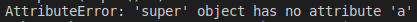Program: Accessing instance variable without super() (demo23.py)

```class P:
def __init__(self):
self.a=20
class C(P):
def m1(self):
print(self.a)

c=C()
c.m1()```

Output: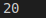###### CASE2:

By using super() function we can access parent class static variables. An example for this is already shown in demo18.py.

Program: Accessing static variable super() (demo24.py)

```class P:
a = 10
def m1(self):
print("m1 from Parent class")

class C(P):
def m2(self):
print(super().a)

c=C()
c.m2()```

Output: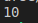###### CASE3:

From child class constructor, we can access parent class

1. instance method
2. static method
3. class method

Program: demo25.py

```class P:
def __init__(self):
print('Parent class Constructor')
def m1(self):
print('m1() instance method from Parent class')

@classmethod
def m2(cls):
print('m2() class method from Parent class')
@staticmethod
def m3():
print('m3() static method from Parent class')
class C(P):
def __init__(self):
super().__init__()
super().m1()
super().m2()
super().m3()
c=C()```

Output: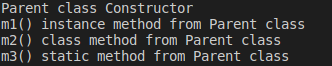From child class instance method, we can access parent class

1. instance method
2. static method
3. class method
###### Program: demo26.py
```class P:
def __init__(self):
print('Parent class Constructor')
def m1(self):
print('m1() instance method from Parent class')

@classmethod
def m2(cls):
print('m2() class method from Parent class')
@staticmethod
def m3():
print('m3() static method from Parent class')
class C(P):
def __init__(self):
print("Child class constructor")
def m1(self):
super().__init__()
super().m1()
super().m2()
super().m3()
c=C()
c.m1()```

Output: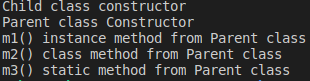###### CASE4:

By using super() function we cannot access parent class instance method and constructor from child class, classmethod. In clear terms, “Parent class – Constructor, Instance Methods” cannot be accessed from “Child class – ClassMethod”

Program: demo27.py

```class P:
def __init__(self):
print('Parent Constructor')
def m1(self):
print('Parent instance method')
@classmethod
def m2(cls):
print('Parent class method')
@staticmethod
def m3():
print('Parent static method')
class C(P):
@classmethod
def m1(cls):
super().__init__()
super().m1()

C.m1()```

Output: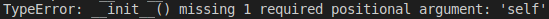Since we are calling a class method, object creation is not required. We discussed about this in the class methods topic of previous chapter. So, there is no object instance for the class, which is by default expected by the constructor and instance methods. Hence, the error.

###### CASE5:

By using super() function we can access parent class static method and class method from child class, class method. In clear terms, “Parent class – Static Method, Class Method” can be accessed from “Child class – ClassMethod”.

Program: demo28.py

```class P:
def __init__(self):
print('Parent Constructor')
def m1(self):
print('Parent instance method')
@classmethod
def m2(cls):
print('Parent class method')
@staticmethod
def m3():
print('Parent static method')
class C(P):
@classmethod
def m1(cls):
super().m2()
super().m3()

C.m1()```

Output: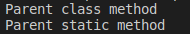###### CASE6:

As discussed in case 4, “Parent class – Constructor, Instance Methods” cannot be accessed from “Child class – ClassMethod”. But there is way to access them.

Program: demo29.py

```class P:
def __init__(self):
print('Parent Constructor')
def m1(self):
print('Parent instance method')
@classmethod
def m2(cls):
print('Parent class method')
@staticmethod
def m3():
print('Parent static method')
class C(P):
@classmethod
def m1(cls):
super(C, cls).__init__(cls)
super(C, cls).m1(cls)

C.m1()```

Output: